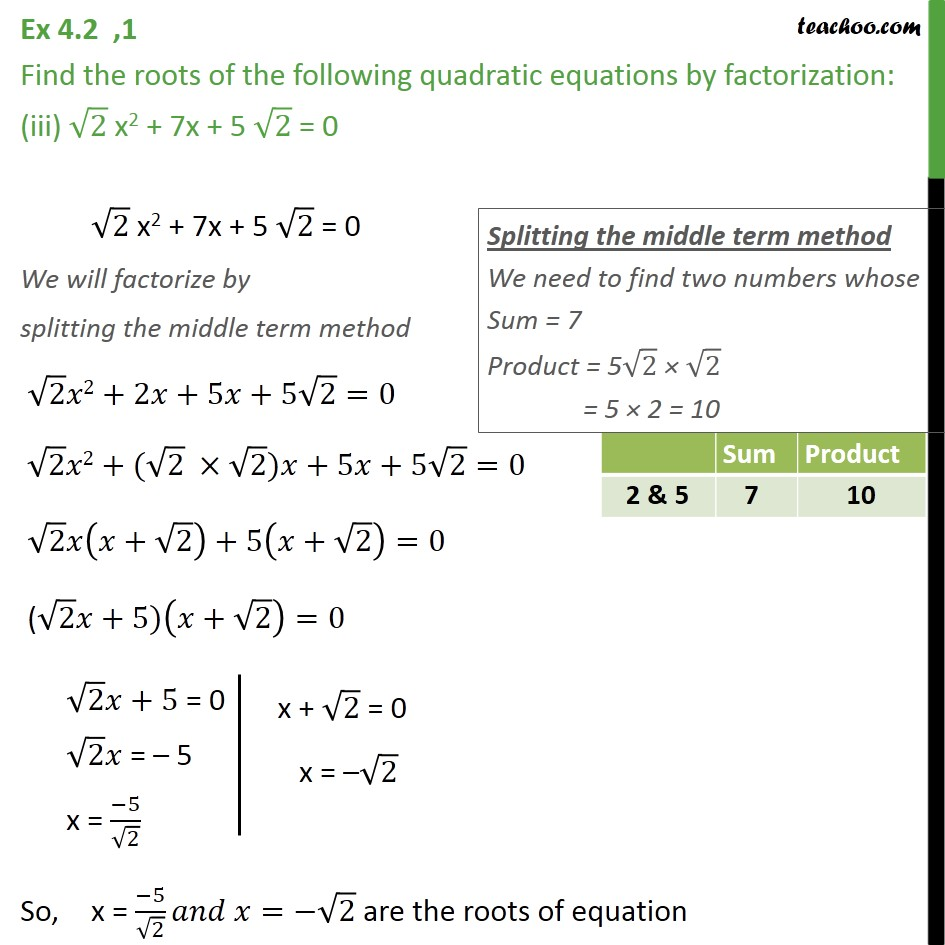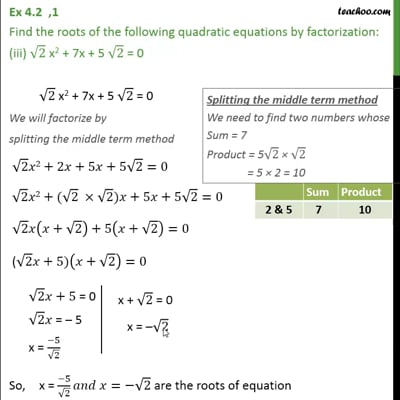Ex 4.2

Chapter 4 Class 10 Quadratic Equations
Serial order wiseThis video is only available for Teachoo black users

Introducing your new favourite teacher - Teachoo Black, at only ₹83 per month

### Transcript

Ex 4.2 ,1 Find the roots of the following quadratic equations by factorization: (iii) 2 x2 + 7x + 5 2 = 0 2 x2 + 7x + 5 2 = 0 We will factorize by splitting the middle term method 2 2+2 +5 +5 2=0 2 2+( 2 2) +5 +5 2=0 2 ( + 2)+5( + 2)=0 ( 2 +5)( + 2)=0 So, x = ( 5)/ 2 = 2 are the roots of equation